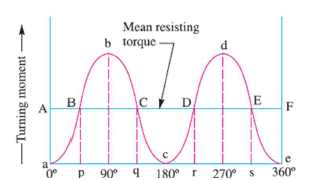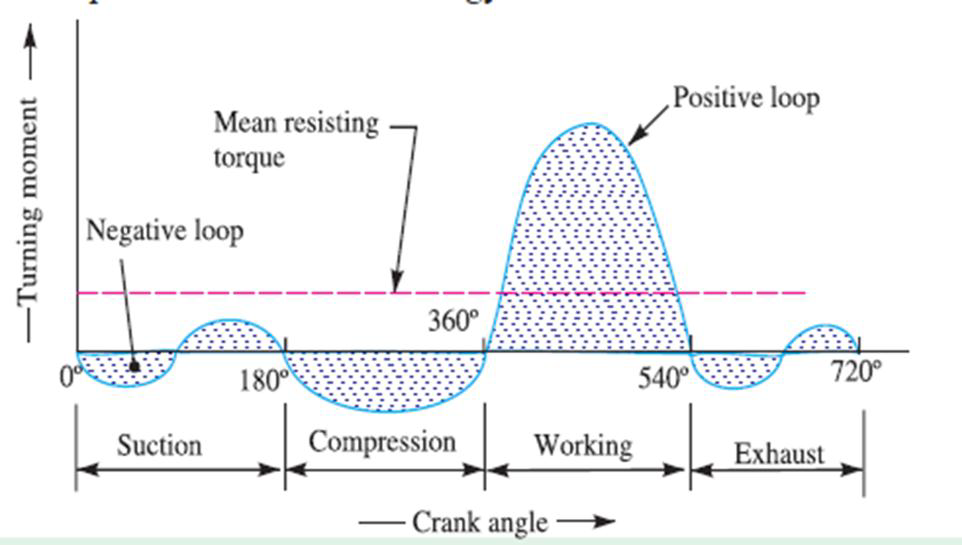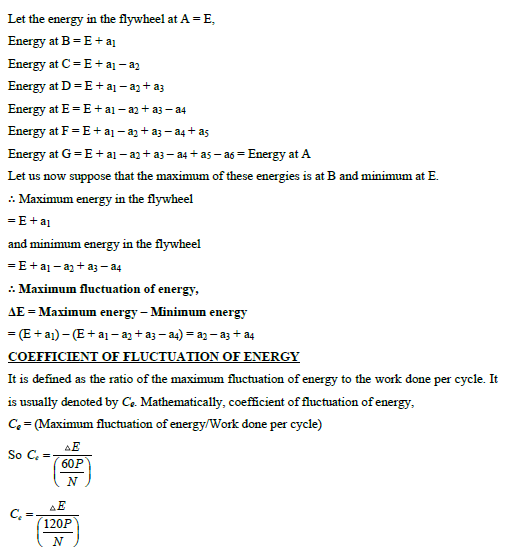Contents

# DESIGN OF FLYWHEEL

INTRODUCTION

A flywheel used in machines serves as a reservoir which stores energy during the period

when the supply of energy is more than the requirement and releases it during

the period when the requirement of energy is more than supply.

## FLYWHEEL VS GOVERNOR

### Flywheel

In machines where the operation is intermittent like punching machines, shearing machines, riveting machines, crushers etc., the flywheel stores energy from the power source during the greater portion of the operating cycle and gives it up during a small period of the cycle. Thus the energy from the power source to the machines is supplied practically at a constant rate throughout the operation.

### Governor

The function of a governor in engine is entirely different from that of a flywheel. It regulates the mean speed of an engine when there are variations in the load, e.g. when the load on the engine increases, it becomes necessary to increase the supply of working fluid. On the other hand, when the load
decreases, less working fluid is required.

The governor automatically controls the supply of working fluid to the engine with the varying load condition and keeps the mean speed within certain limits.

## FLUCTUATION OF ENERGY

The fluctuation of energy may be determined by the turning moment diagram for one complete cycle of operation. Consider a turning moment diagram for a single cylinder double acting steam engine as shown in Fig. . The vertical ordinate represents the turning moment and the horizontal ordinate (abscissa) represents the crank angle. A little consideration will show that the turning moment is zero when the crank angle is zero. It rises to a maximum value when crank angle reaches 90º and it is again zero when crank angle is 180º. This is shown by the curve abc in Fig.  and it represents the turning moment diagram for outstroke. The curve cde is the turning moment diagram for instroke and is somewhat similar to the curve abc. Since the work done is the product of the turning moment and the angle turned, therefore the area of the turning moment diagram represents the work done per revolution. In actual practice, the engine is assumed to work against the mean resisting torque, as shown by a horizontal line AF. The height of the ordinate A represents the mean height of the turning moment diagram. Since it is assumed that the work done by the turning moment per revolution is equal to the work done against the mean resisting torque, therefore the area of the rectangle aAFe is proportional to the work done against the mean resisting torque.Turning moment diagram of single cylinder double acting steam engine

It can be seen in Figure, that the mean resisting torque line AF cuts the turning moment diagram at points B, C, D and E. When the crank moves from ‘a’ to ‘p’ the work done by the engine is equal to the area aBp, whereas the energy required is represented by the area aABp. In other words, the engine has done less work (equal to the area aAB) than the requirement. This amount of energy is taken from the flywheel and hence the speed of the flywheel decreases. Now the crank moves from p to q, the work done by the engine is equal to the area pBbCq, whereas the requirement of energy is represented by the area pBCq. Therefore the engine has done more work than the requirement. This excess work (equal to the area BbC) is stored in the flywheel and hence the speed of the flywheel increases while the crank moves from p to q. Similarly when the crank moves from q to r, more work is taken from the engine than is developed. This loss of work is represented by the area CcD. To supply this loss, the flywheel gives up some of its energy and thus the speed decreases while the crank moves from q to r. As the crank moves from r to s, excess energy is again developed given by the area DdE and the speed again increases. As the piston moves from s to e, again there is a loss of work and the speed decreases. The variations of energy above and below the mean resisting torque line are called fluctuation of energy. The areas BbC, CcD, DdE etc. represent fluctuations of energy. A turning moment diagram for a four stroke internal combustion engine is shown in FigureTurning moment diagram for a four stroke internal combustion engine

## MAXIMUM FLUCTUATION OF ENERGY

A turning moment diagram for a multi-cylinder engine is shown by a wavy curve in Fig. . The horizontal line AG represents the mean torque line. Let a1, a3, a5 be the areas above the mean torque line and a2, a4 and a6 be the areas below the mean torque line. These areas represent some quantity of energy which is either added or subtracted from the energy of the moving parts of the engine.## ENERGY STORED IN A FLYWHEEL

A flywheel is shown in Fig. . It has already been discussed that when a flywheel absorbs
energy its speed increases and when it gives up energy its speed decreases.